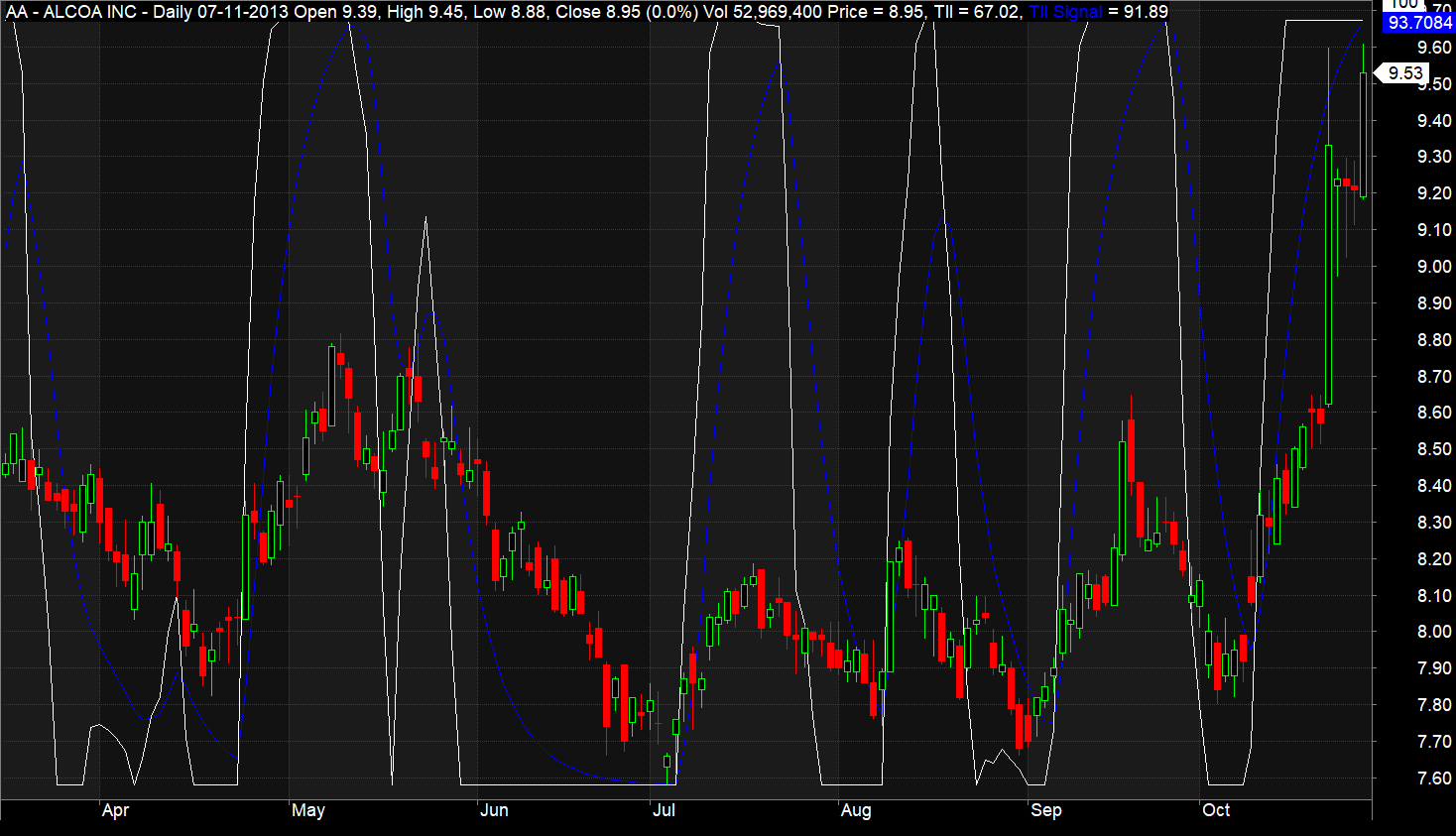# Trend Intensity Index (TII) AFL Code

Trend Intensity Index (TII) is an oscillator that measures the strength of the current trend for any financial instrument. It can be used independently or in conjunction with other trend-following indicators to accurately gauge the price movements during trending markets.

Developed by M.H Pee, TII oscillates between 0 to 100%. A value above 80% is an indicator of a strong bullish trend, while a value below 20% is an indicator of a strong bearish trend.

The indicator also consists of a signal line which is 9 periods exponential moving average of the TII line.

## Preferred Timeframe

You can use this indicator in any timeframe.

Also Read: Know Sure Thing (KST) AFL Code

## Screenshot

Below is the screenshot of the trend intensity index (TII) indicator plotted in an Amibroker chart. It is plotted as a white line over the candlestick chart.

Along with TII, a signal line is also plotted as a blue line.## AFL Explanation

The calculation of Trend intensity index is a multi-step process as described below:

Step 1: Calculate the simple moving average of close price for ‘n’ periods. Call it SMA

Step 2: Find out the positive and negative deviation of the close price at each candle with respect of SMA calculated in the previous step

Dev = Close – SMA

If (Dev>0) then posDev=Dev, else negDev=abs(Dev)

Step 3: Calculate the sum of Positive and Negative deviations for the period twice smaller than the period used for SMA calculation

SDpos = sum(posDev, m);

SDneg = sum(negDev, m);

Here m = n/2 if n is even number and (n+1)/2 if n is odd number

Step 4: Calculate trend intensity index using the below formula

TII = 100 * (SDpos) / (SDpos + SDneg);

Step 5: Calculate signal line as a 9 periods exponential moving average of trend intensity index

TIISignal = EMA(TII, 9);

And then, TII and TIISignal are plotted on a candlestick chart by using the standard Amibroker “plot” function.

Do you want to learn AFL coding from scratch and build your own profitable trading systems? Check out our best-selling Algorithmic trading Bootcamp course bundle.

## Long and Short Rules

Here are the long and short rules you can consider while trading through TRIMA:

1. If TII is above 80, it signifies strong
2. If TII is below 20, it signifies strong downtrend
3. If TII rises above 20, go long
4. If TII goes below 80, go short
5. If TII crosses above TIISignal, go long
6. If TII crosses below TIISignal, go short

Disclaimer:

All the AFL’s posted in this section are for learning purposes. Trading Tuitions does not necessarily own these AFL’s and we don’t have any intellectual property rights on them. We might copy useful AFL’s from public forums and post it in this section in a presentable format. The intent is not to copy anybody’s work but to share knowledge. If you find any misleading or non-reproducible content then please inform us at support@tradingtuitions.com